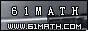Today is . WELCOME: huohai3983/44663  Home | Reg Login | All user | setHomePage | BookmarkHome | Math Test Online | Addition | Subtraction | Multiplication | Division | Time | Worksheet(JPG/PDF) | CAI | Flash Games |
 No. Sorted by Grade Level Kindergarten   First Grade    Second Grade    Third Grade    Fourth Grade 1Addition and Subtraction of Decimals [Results] /45612 2Place Value [Results] /63812 3Multiplying two digit by one digit numbers(Less Than 100) [Results] /32748 4Multiplication of Tens and one digit numbers [Results] /22649 5Multiplying three digit by one two numbers [Results] /33845 6Division of Tens [Results] /18312 7Divide a Hundreds by Tens [Results] /17443 8Divide a 3 Digit by Tens [Results] /19127 9Divide a 3 Digit two digit numbers [Results] /33663 10Decimals [Results] /18068 11Addition and Subtraction of Decimals [Results] /28091 Sorted by Grade Level Kindergarten First | Second | Third | FourthGreatmathsites

### Translate

 Thank you to online math channels' Mathematics Department for hosting these lessons. Copyright © 2006 - 2008 GZtrain Inc. All Rights Reserved